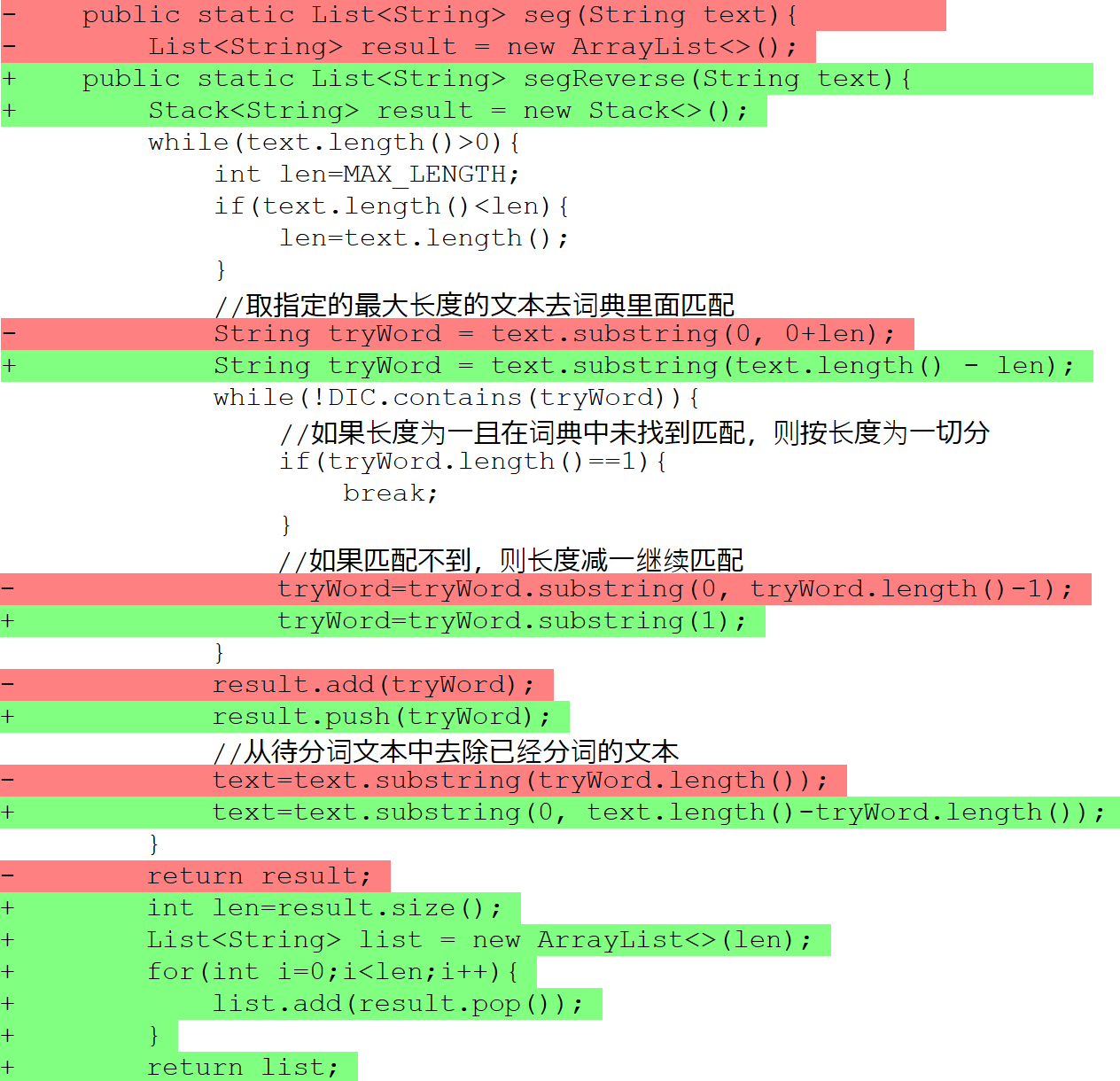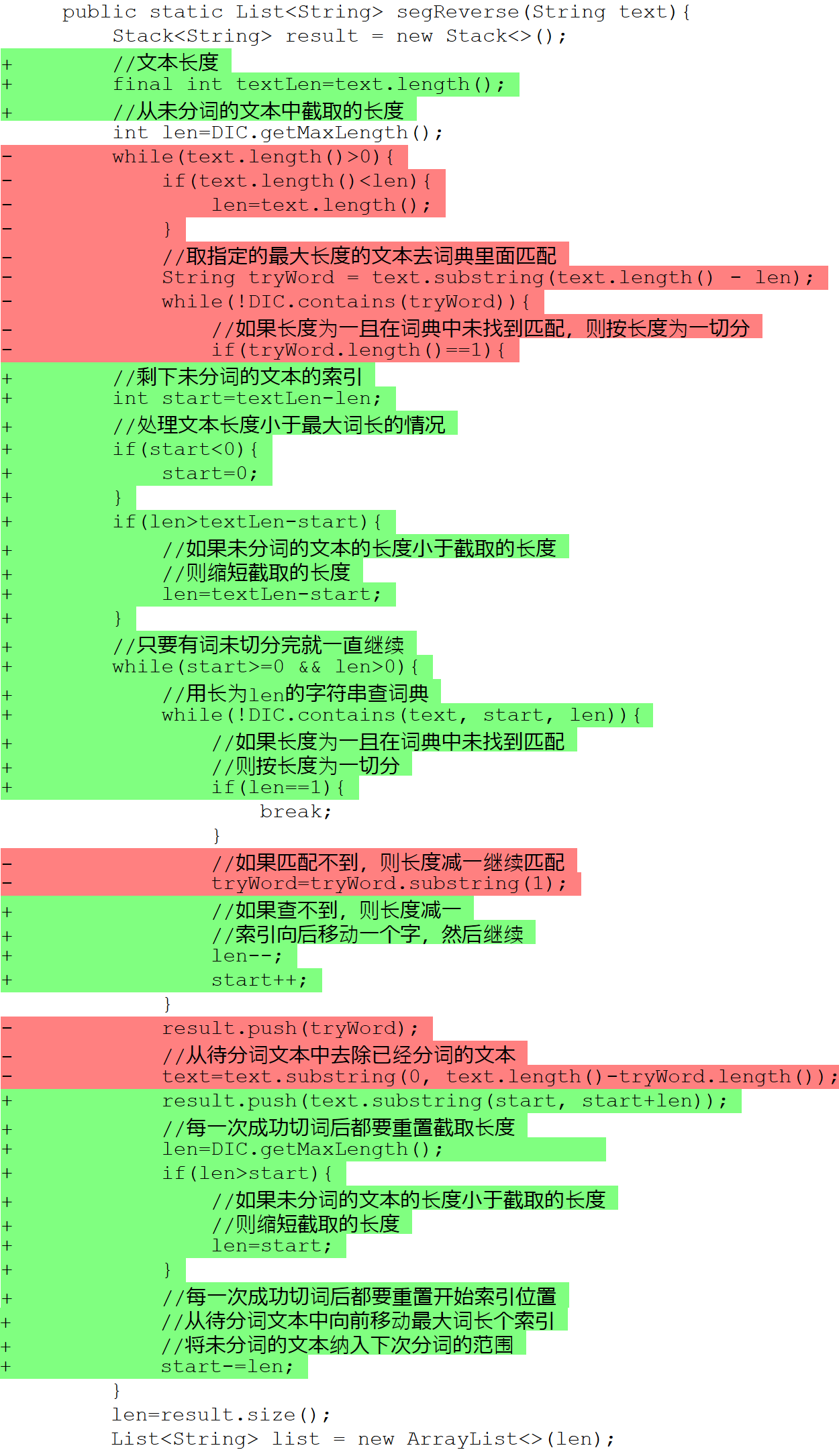# python双向最大匹配算法_中文分词算法 之 基于词典的逆向最大匹配算法

public static List segReverse(String text){

Stack result = new Stack<>();

while(text.length()>0){

int len=MAX_LENGTH;

if(text.length()

len=text.length();

}

//取指定的最大长度的文本去词典里面匹配

String tryWord = text.substring(text.length() - len);

while(!DIC.contains(tryWord)){

//如果长度为一且在词典中未找到匹配，则按长度为一切分

if(tryWord.length()==1){

break;

}

//如果匹配不到，则长度减一继续匹配

tryWord=tryWord.substring(1);

}

result.push(tryWord);

//从待分词文本中去除已经分词的文本

text=text.substring(0, text.length()-tryWord.length());

}

int len=result.size();

List list = new ArrayList<>(len);

for(int i=0;i

list.add(result.pop());

}

return list;

}public static void main(String[] args){

List sentences = new ArrayList<>();

sentences.add("杨尚川是APDPlat应用级产品开发平台的作者");

sentences.add("研究生命的起源");

sentences.add("长春市长春节致辞");

sentences.add("他从马上下来");

sentences.add("乒乓球拍卖完了");

sentences.add("咬死猎人的狗");

sentences.add("大学生活象白纸");

sentences.add("他有各种才能");

sentences.add("有意见分歧");

for(String sentence : sentences){

System.out.println("正向最大匹配: "+seg(sentence));

System.out.println("逆向最大匹配: "+segReverse(sentence));

}

}

/**

* 将一个文件分词后保存到另一个文件

* @author 杨尚川

*/

public class SegFile {

public static void main(String[] args) throws Exception{

String input = "input.txt";

String output = "output.txt";

if(args.length == 2){

input = args;

output = args;

}

long start = System.currentTimeMillis();

segFile(input, output);

long cost = System.currentTimeMillis()-start;

System.out.println("cost time:"+cost+" ms");

}

public static void segFile(String input, String output) throws Exception{

float max=(float)Runtime.getRuntime().maxMemory()/1000000;

float total=(float)Runtime.getRuntime().totalMemory()/1000000;

float free=(float)Runtime.getRuntime().freeMemory()/1000000;

String pre="执行之前剩余内存:"+max+"-"+total+"+"+free+"="+(max-total+free);

try(BufferedReader reader = new BufferedReader(new InputStreamReader(new FileInputStream(input),"utf-8"));

BufferedWriter writer = new BufferedWriter(new OutputStreamWriter(new FileOutputStream(output),"utf-8"))){

int textLength=0;

long start = System.currentTimeMillis();

String line = reader.readLine();

while(line != null){

textLength += line.length();

writer.write(WordSeg.seg(line).toString()+"\n");

line = reader.readLine();

}

long cost = System.currentTimeMillis() - start;

float rate = textLength/cost;

System.out.println("文本字符："+textLength);

System.out.println("分词耗时："+cost+" 毫秒");

System.out.println("分词速度："+rate+" 字符/毫秒");

}

max=(float)Runtime.getRuntime().maxMemory()/1000000;

total=(float)Runtime.getRuntime().totalMemory()/1000000;

free=(float)Runtime.getRuntime().freeMemory()/1000000;

String post="执行之后剩余内存:"+max+"-"+total+"+"+free+"="+(max-total+free);

System.out.println(pre);

System.out.println(post);

}

}

dic.class=org.apdplat.word.dictionary.impl.TrieV3

dic.path=dic.txt

cost time:64029 ms

dic.class=org.apdplat.word.dictionary.impl.HashSet

dic.path=dic.txt

cost time:77271 ms

public static List seg(String text){

List result = new ArrayList<>();

while(text.length()>0){

int len=MAX_LENGTH;

if(text.length()

len=text.length();

}

//取指定的最大长度的文本去词典里面匹配

String tryWord = text.substring(0, 0+len);

while(!DIC.contains(tryWord)){

//如果长度为一且在词典中未找到匹配，则按长度为一切分

if(tryWord.length()==1){

break;

}

//如果匹配不到，则长度减一继续匹配

tryWord=tryWord.substring(0, tryWord.length()-1);

}

result.add(tryWord);

//从待分词文本中去除已经分词的文本

text=text.substring(tryWord.length());

}

return result;

}

public static List seg(String text){

List result = new ArrayList<>();

//文本长度

final int textLen=text.length();

//从未分词的文本中截取的长度

int len=DIC.getMaxLength();

//剩下未分词的文本的索引

int start=0;

//只要有词未切分完就一直继续

while(start

if(len>textLen-start){

//如果未分词的文本的长度小于截取的长度

//则缩短截取的长度

len=textLen-start;

}

//用长为len的字符串查词典

while(!DIC.contains(text, start, len)){

//如果长度为一且在词典中未找到匹配

//则按长度为一切分

if(len==1){

break;

}

//如果查不到，则长度减一后继续

len--;

}

result.add(text.substring(start, start+len));

//从待分词文本中向后移动索引，滑过已经分词的文本

start+=len;

//每一次成功切词后都要重置截取长度

len=DIC.getMaxLength();

}

return result;

}

public static List segReverse(String text){

Stack result = new Stack<>();

//文本长度

final int textLen=text.length();

//从未分词的文本中截取的长度

int len=DIC.getMaxLength();

//剩下未分词的文本的索引

int start=textLen-len;

//处理文本长度小于最大词长的情况

if(start<0){

start=0;

}

if(len>textLen-start){

//如果未分词的文本的长度小于截取的长度

//则缩短截取的长度

len=textLen-start;

}

//只要有词未切分完就一直继续

while(start>=0 && len>0){

//用长为len的字符串查词典

while(!DIC.contains(text, start, len)){

//如果长度为一且在词典中未找到匹配

//则按长度为一切分

if(len==1){

break;

}

//如果查不到，则长度减一

//索引向后移动一个字，然后继续

len--;

start++;

}

result.push(text.substring(start, start+len));

//每一次成功切词后都要重置截取长度

len=DIC.getMaxLength();

if(len>start){

//如果未分词的文本的长度小于截取的长度

//则缩短截取的长度

len=start;

}

//每一次成功切词后都要重置开始索引位置

//从待分词文本中向前移动最大词长个索引

//将未分词的文本纳入下次分词的范围

start-=len;

}

len=result.size();

List list = new ArrayList<>(len);

for(int i=0;i

list.add(result.pop());

}

return list;

}

dic.class=org.apdplat.word.dictionary.impl.TrieV3

dic.path=dic.txt

cost time:25007 ms

dic.class=org.apdplat.word.dictionary.impl.HashSet

dic.path=dic.txt

cost time:40928 ms

public class HashSet implements Dictionary{

private Set set = new java.util.HashSet<>();

private int maxLength;

@Override

public int getMaxLength() {

return maxLength;

}

@Override

public boolean contains(String item, int start, int length) {

return set.contains(item.substring(start, start+length));

}

@Override

public boolean contains(String item) {

return set.contains(item);

}

@Override

public void addAll(List items) {

for(String item : items){

add(item);

}

}

@Override

public void add(String item) {

//去掉首尾空白字符

item=item.trim();

int len = item.length();

if(len

//长度小于1则忽略

return;

}

if(len>maxLength){

maxLength=len;

}

set.add(item);

}

}

JDK的HashSet没有这里优化所使用的contains(String item, int start, int length)方法，所以用了substring，这是HashSet提速没有TrieV3大的原因之一。10-2012-0321
11-2364
01-08749
11-23333
12-0538
08-221960
01-271万+
10-284784
03-20284
05-25410
10-24716
02-182486
03-231211
02-091105
05-281万+
©️2020 CSDN 皮肤主题: 游动-白 设计师:白松林